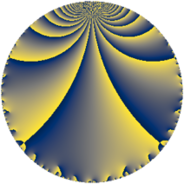# Properties

 Label 38.3.dLevel $38$ Weight $3$ Character orbit 38.d Rep. character $\chi_{38}(27,\cdot)$ Character field $\Q(\zeta_{6})$ Dimension $4$ Newform subspaces $1$ Sturm bound $15$ Trace bound $0$

# Related objects

## Defining parameters

 Level: $$N$$ $$=$$ $$38 = 2 \cdot 19$$ Weight: $$k$$ $$=$$ $$3$$ Character orbit: $$[\chi]$$ $$=$$ 38.d (of order $$6$$ and degree $$2$$) Character conductor: $$\operatorname{cond}(\chi)$$ $$=$$ $$19$$ Character field: $$\Q(\zeta_{6})$$ Newform subspaces: $$1$$ Sturm bound: $$15$$ Trace bound: $$0$$

## Dimensions

The following table gives the dimensions of various subspaces of $$M_{3}(38, [\chi])$$.

Total New Old
Modular forms 24 4 20
Cusp forms 16 4 12
Eisenstein series 8 0 8

## Trace form

 $$4q + 6q^{3} + 4q^{4} + 2q^{5} - 4q^{6} + 8q^{7} - 8q^{9} + O(q^{10})$$ $$4q + 6q^{3} + 4q^{4} + 2q^{5} - 4q^{6} + 8q^{7} - 8q^{9} - 40q^{11} - 30q^{13} - 24q^{14} + 6q^{15} - 8q^{16} + 14q^{17} + 16q^{19} + 8q^{20} + 36q^{21} + 24q^{22} - 10q^{23} + 8q^{24} + 48q^{25} + 48q^{26} + 8q^{28} + 66q^{29} - 8q^{30} - 84q^{33} + 24q^{34} + 4q^{35} + 16q^{36} - 84q^{38} - 108q^{39} + 18q^{41} - 32q^{42} + 38q^{43} - 40q^{44} - 16q^{45} - 70q^{47} - 24q^{48} - 84q^{49} + 18q^{51} - 60q^{52} - 42q^{53} - 28q^{54} - 20q^{55} + 102q^{57} + 144q^{58} + 42q^{59} + 12q^{60} + 74q^{61} + 32q^{63} - 32q^{64} + 64q^{66} + 102q^{67} + 56q^{68} - 24q^{70} + 306q^{71} + 48q^{72} + 98q^{73} + 72q^{74} + 4q^{76} - 176q^{77} + 132q^{78} - 126q^{79} + 8q^{80} + 10q^{81} - 168q^{82} - 64q^{83} - 14q^{85} - 276q^{86} - 12q^{87} - 6q^{89} - 24q^{90} - 204q^{91} + 20q^{92} - 108q^{93} + 14q^{95} + 32q^{96} - 486q^{97} - 96q^{98} + 32q^{99} + O(q^{100})$$

## Decomposition of $$S_{3}^{\mathrm{new}}(38, [\chi])$$ into newform subspaces

Label Dim. $$A$$ Field CM Traces $q$-expansion
$$a_2$$ $$a_3$$ $$a_5$$ $$a_7$$
38.3.d.a $$4$$ $$1.035$$ $$\Q(\sqrt{-2}, \sqrt{-3})$$ None $$0$$ $$6$$ $$2$$ $$8$$ $$q+\beta _{1}q^{2}+(1-\beta _{1}+\beta _{2})q^{3}+2\beta _{2}q^{4}+\cdots$$

## Decomposition of $$S_{3}^{\mathrm{old}}(38, [\chi])$$ into lower level spaces

$$S_{3}^{\mathrm{old}}(38, [\chi]) \cong$$ $$S_{3}^{\mathrm{new}}(19, [\chi])$$$$^{\oplus 2}$$